﻿ Diffusion Coefficient Estimation in Shrinking Solids. A Case Study: Tomato

Diffusion Coefficient Estimation in Shrinking Solids. A Case Study: Tomato

C. Martínez-Vera, I. Anaya-Sosa, M. G. Vizcarra-Mendoza

American Journal of Food Science and Technology

Diffusion Coefficient Estimation in Shrinking Solids. A Case Study: Tomato

C. Martínez-Vera1,, I. Anaya-Sosa2, M. G. Vizcarra-Mendoza1

1Departamento de Ingeniería de Procesos e Hidráulica, Universidad Autónoma Metropolitana-Iztapalapa.Apartado Postal 55-534, México D.F., 09340, México

2Escuela Nacional de Ciencias Biológicas, Instituto Politécnico Nacional. Unidad Profesional Lázaro Cárdenas, Prolongación de Carpio y Plan de Ayala s/n, Col. Santo Tomas C.P. 11340. Del. Miguel Hidalgo. México, D.F

Abstract

In this work are presented experimental results of the drying kinetics for a solid that shrinks between 88% and 94% in volume during the drying process depending on the drying temperature. The drying process is modeled and simulated as an isothermal diffusional process taking in account the shrinkage of the dried material. Two falling-rate drying periods were considered in the drying process. Diffusion-moisture content relationships were determined for each drying period at each of the temperature levels at which the experiments were conducted. Arrhenius type expressions were obtained for the diffusion-temperature dependency from the diffusion-moisture content profiles averaged at each drying temperature considered in this study for each drying period.

• C. Martínez-Vera, I. Anaya-Sosa, M. G. Vizcarra-Mendoza. Diffusion Coefficient Estimation in Shrinking Solids. A Case Study: Tomato. American Journal of Food Science and Technology. Vol. 3, No. 5, 2015, pp 132-136. http://pubs.sciepub.com/ajfst/3/5/3
• Martínez-Vera, C., I. Anaya-Sosa, and M. G. Vizcarra-Mendoza. "Diffusion Coefficient Estimation in Shrinking Solids. A Case Study: Tomato." American Journal of Food Science and Technology 3.5 (2015): 132-136.
• Martínez-Vera, C. , Anaya-Sosa, I. , & Vizcarra-Mendoza, M. G. (2015). Diffusion Coefficient Estimation in Shrinking Solids. A Case Study: Tomato. American Journal of Food Science and Technology, 3(5), 132-136.
• Martínez-Vera, C., I. Anaya-Sosa, and M. G. Vizcarra-Mendoza. "Diffusion Coefficient Estimation in Shrinking Solids. A Case Study: Tomato." American Journal of Food Science and Technology 3, no. 5 (2015): 132-136.

 Import into BibTeX Import into EndNote Import into RefMan Import into RefWorks

12
Prev Next

1. Introduction

Modeling the drying of shrinking solids as a fickian diffusional process presents some challenges. Taking in account the volume reduction increases the ill-conditioning of the non-linear equations that describe the process. The diffusion coefficient determination for cases as the one here considered are an open problem. Sometimes the diffusion coefficients are determined neglecting the shrinking effect (Demiray & Tulek, 2012 ). Other times the diffusion coefficients are determined departing from a mathematical solution of the problem not rigorously valid for the case considered: a truncated Fourier series solution in which the shrinking effect is included forcing the solution, and the diffusion coefficient to follow the experimental data (Hawlader, 2002 ; Giovanelli et al., 2002 ; Resende et al., 2007 ). Here we follow another approach: the drying-shrinking process is modeled rigorously taking in account the shrinkage and assuming a diffusion coefficient dependent on moisture content (Crank, 1975 ; Crank, 1984 ; Aguerre et al., 2008 ). The diffusion coefficient to be determined is an effective one that involves all moisture transport mechanisms that could be involved in the drying process. The moving boundary problem is transformed in a fixed boundary problem through a change of variable (Crank, 1975 ). The solids chosen as a case study are peeled tomato cubes. Tomato is a foodstuff with high moisture content that when is being dried presents a high volume reduction due to the water lost. A set of experiments were performed for drying of tomato mesocarp cut into cubes isothermically and the evolution of the moisture content was registered at several temperatures. The equilibrium moisture contents at the solid’s surface were determined experimentally at each of the temperature levels analyzed in this study. The proper diffusion-moisture content relationship was determined fitting the parameters of the diffusion coefficient model assumed in order to give a good agreement with the experimental results by a trial and error procedure. The experimental and simulated drying kinetics curves and the solid’s volume time evolution are presented for drying at 60°C, 70°C and 80°C in a moisture balance.

The main goal of the present study was to calculate the effective moisture diffusivity for the tomato mesocarp not departing from a solution to the diffusion equation no longer valid when the schrinking effect is taken in account but from a numerical solution to that equation when the volume reduction is considered. The activation energy was also evaluated.

2. Materials and Methods

2.1. Experimental

Fresh, mature and ripe red tomatoes (Lycopersicum Esculentum, cv. Roma) were purchased from a local market in México City. All samples were chosen as the same size and color. The tomatoes were washed and several longitudinal cuts were done along their surfaces. The whole tomatoes were dipped in a 0.026% by weight CaCl2 solution at 75°C for 60 s. After this treatment the tomatoes were peeled, the endocarp was eliminated by hand and the mesocarp (the fleshy part of the pericarp) was cut in cubes 1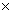10.7 cm3. The initial moisture content of the tomato cubes was determined by the oven drying method, drying the solids in an oven at 95°C for 3 hours and, by gravimetry, the moisture content was found to be between 93 to 96% wb (Rivera, 2007 ). The dry mass was determined according to the methodology proposed by Lewicki (2002)  consisting in drying a sample in an oven at 95°C for 3 hours. The reported sphericity of cubes is 0.81 (Kunii & Levenspiel, 1991 ), which was confirmed by the oil displacement method). Drying experiments were performed in a moisture balance (Ohaus, MB200) with an accuracy of 0.01 g at three temperature levels: 60°C, 70°C and 80°C for 10800 s.

For modeling purposes the cubes were considered as spheres due to the fact that the cubes were rapidly deformed and converted in amorphous solids whose change of form could not be followed and whose superficial area is difficult to determine. Volume changes of individual samples were determined by the method of organic oil displacement in a graduated probet and from this volume the radius of the equivalent sphere was determined (initially it was 6.1010-3 m). All the drying experiments were repeated twice. Each experimental run and it´s duplicate were not significantly different at 0.05 confidence level.

2.2. Mathematical Modeling

The solid particles are modeled as if they were spheres with moisture content gradients only in the radial direction. Assuming that the drying process is described by the diffusion equation (with an effective diffusivity that takes in account all possible moisture transport mechanisms) and that the solid volume reduction is proportional to the volume of water lost by the solid we describe the drying and shrinking of a solid particle under isothermal conditions taking in account the dependency of the volumetric moisture content on the time and volume variables and the dependency of the radius with time (Crank, 1975 ; Aguerre et al., 2008 ). The moving boundary problem is transformed into a fixed boundary problem through a variable change (z=r/R) in order to have variations of the dimensionless spatial variable between 0 and 1. The isothermicity condition leads to a Dirichlet condition at the border and the consideration of a spherical particle leads to a symmetry condition at the center of the particle. The resulting equations in terms of the volumetric moisture concentration, c, and dimensionless radial position are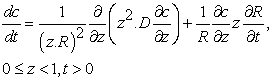(1a)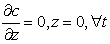(1b)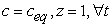(1c)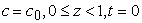(1d)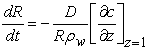(1e)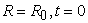(1f)(1g)

where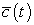denotes the volumetric moisture content averaged over the volume at each time step and the diffusion coefficient-moisture content relationship is assumed to be of the next form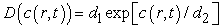(2)

where the parameters d1 and d2 are fitting parameters to be determined for each experimental temperature.

The above given equations that describe the isothermal drying process (1a-1g) were solved discretizing the spatial variable by the finite differences technique (with a 160 points mesh) and the resulting system of non-linear ordinary differential equations together with the differential equation that describes the radius evolution (1e) were integrated by a proper method for stiff equations (Rosenbrock method (Press et al., 2007 )). The spatial moisture profiles inside the particle were numerically averaged at each time step (Equation (1g)) for obtaining the average moisture profile at each time step.

In order to fit the parameters of the diffusion-moisture model through a comparison of the average moisture content profile with the corresponding experimental profile at each level of temperature the experimental moisture content data (db) at each temperature were fitted by an exponential function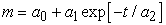(3)

where a0 , a1 and a2 are the parameters to be fitted by a.

3. Results and Discussion

Table 1. Initial and equilibrium moisture contents and dry masses

The experimental drying curves were obtained and the reduction in size was registered (the initial radius R0 = 6.1010-3 m and the final radiuses were: 2.9610-3 m at 60°C, 2.6510-3 m at 70°C and 2.4110-3 m at 80°C). In Table 1 are reported the initial moisture content, the equilibrium moisture content and the solid’s dry mass corresponding to each drying temperature. The experimental drying curves (db) in dimensionless form for drying at 60°C, 70°C and 80°C (the corresponding initial moisture contents were 93.90, 94.70 and 94.99 % wb at each temperature) are shown in Figure 1. In Figure 2 are shown the drying rate versus moisture content (db) curves. No constant rate period was observed in the drying of tomato mesocarp cubes. It can be observed that the drying rates are higher at higher temperatures and also that at 70°C and 80°C there are at least two drying periods and that at the lower temperature, 60°C, there are only one in the range of moisture content analyzed.Download asVeiw figureFigures index
Figure 1. Experimental moisture content values (symbols) and simulated drying curves (continuous lines) obtained solving Equations (1a-1g) and Equation (2).

The parameters a0, a1 and a2 that fit equation (3) to the experimental moisture content data (db) for drying at 60°C, 70°C and 80°C are given in Table 2. In all cases the regression coefficients were 0.999.Download asVeiw figureFigures index
Figure 2. Drying rate versus moisture content

In order to obtain an acceptable agreement between the predicted average moisture content (simulation results) and the experimental data the constant d1 had to be adjusted as a step function for each temperature level changing abruptly at certain critical time (which corresponds to certain critical moisture content). It was not possible to obtain an acceptable prediction of the experimental results with a constant diffusion coefficient at each temperature.

Table 2. Constants for the expression fitting the experimental kinetic data

The parameter values for equation (2) at the three temperatures studied are reported in Table 3 where d1l and d1h denote the values of the parameter d1 fitted below and above the critical moisture content at which this parameter changes in a step form. These parameters were adjusted by a trial and error procedure on the program in which equation (1) was solved until a good agreement between the experimental and the simulated drying curves was obtained. The simulated profiles obtained with the parameters reported in Tables 1, 2 and 3 are shown in Figure 1 in which can be appreciated the good agreement among the simulation results (continuous line) and the experimental data (dots). The model predictions for the way in which the radius changes with time at the three temperature levels analyzed are shown in Figure 3.

Table 3. Parameters of the diffusion coefficient model (Equation 2)Download asVeiw figureFigures index
Figure 3. Model predictions of the radius reduction in the drying process

In Figure 4 are shown the profiles of the volumetric moisture content [kg/m3] at the interface which increases continually due to the effect of the reduction in volume. Even though the dry base interfacial moisture content is constant the volumetric moisture content is changing due to the shrinking effect and, at the higher temperature, this effect is much more pronounced.Download asVeiw figureFigures index
Figure 4. Evolution of the volumetric surface moisture content

The spatial averaged diffusion coefficients obtained for an instant of time above and below the moisture content value at which the diffusion changes drastically for each temperature are shown in Figure 5 where can be seen the moisture content corresponding to each temperature and the magnitude of the step change in the averaged diffusion coefficient. This information is summarized in Table 4 in which are reported the spatial average diffusion coefficient values, the moisture content at which they change at each drying temperature as well as the corresponding time.

Table 4. Average Diffusion coefficients and critical moisture contentsDownload asVeiw figureFigures index
Figure 5. Spatial averaged diffusion coefficient versus moisture content.

Average diffusion coefficient values at each temperature level were obtained integrating Equation (2) with respect to the volumetric moisture content and dividing by the range of moisture contents considered (Equation (4)). The moisture content limits were chosen in such a way that the minimum and maximum volumetric moisture content values for each experimental run lay in between them.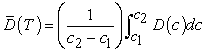(4)

The values of D averaged with respect to volumetric moisture content at each temperature level () obtained with Equation (4) are reported in Table 4. From the graphic of the natural logarithm of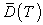nversus 1/T (see Figure 6) for the high and low moisture content ranges (which are defined by the volumetric moisture contents below and above the critical moisture contents reported in Table 4) at each temperature level analyzed are obtained the activation energies and the pre exponential factors corresponding to each drying period postulated in this work. The fitted parameters for the Arrhenius model (Equation 5) leads to an activation energy for moisture contents above than about 12 kg kg-1 (db) Eah = 33.710 kJ mol-1 and a pre exponential factor k0h = 3.10410-7 m2s-1 (R2 = 0.9951) and, for moisture contents below than 12 kg kg-1 (db), Eal = 26.127 kJ mol-1 and a pre exponential factor k0l = 1.67410-8 m2s-1 (R2 = 0.9898).(5)

The moisture diffusivity values reported in the literature for tomato (which could correspond to different geometries, temperatures, experimental conditions, etc.) range from 1.68310-12 m2 s-1 (Kingsly et al., 2007 ) to 2.310-9 m2 s-1 (Giovanelli et al., 2002 ). The values obtained in this work are in the order of those reported by Kingsly et al. (2007) . However it should be taken in account that, as pointed out by Liu et al. (2010) , food shrinkage produces a reduction in the distance required for the movement of water molecules, facilitating the moisture diffusion towards the outer surface and making the effective moisture diffusivity overestimated when the shrinking effect is not taken in account properly. Sometimes the effective diffusion coefficient is determined from the truncated Fourier series solution (for example Doymaz, 2006 ; Resende et al., 2007 ; Abano et al., 2011 ) or from a modification of this solution to the diffusion equation (Giovanelli et al., 2002 ) that is no longer valid when the diffusion coefficient is moisture content dependent or when the shrinking effect cannot be neglected (the Henderson and Pabis model is equivalent to taking in account just the first term of the Fourier series solution above mentioned).Download asVeiw figureFigures index
Figure 6. Fitting Arrhenius’s models to the diffusion coefficients dependency on temperature for the high (spheres) and low (triangles) moisture content ranges at each temperature level analyzed (Eah = 33.710kJ mol-1; Eal = 26.127 kJ mol-1)

The presence of two different diffusion coefficient expressions for each drying air temperature implies two falling rate periods in the drying of pieces of tomato mesocarp. The existence of more than one falling rate drying period has been reported by other authors in drying some agricultural products. Chaijan et al. (2011)  found two drying falling-rate periods in the drying of high moisture corn. These authors reported expressions for the effective moisture diffusivity for each drying period at different air velocities. Taiwo et al. (2006)  found three falling rate periods in their study of drying of tomato slices. Also Hawlader et al. (1991)  reported two falling rate periods in the drying of tomato slices.

The results indicate that at the three temperature levels analyzed the diffusion coefficient is a weak function of moisture content (the diffusion-moisture content profiles appear as nearly constant at each temperature in the range above and below the critical moisture content) and it depends more strongly on temperature as can be appreciated in Figure 5. This fact could justify that the diffusion coefficient dependence on moisture content could be neglected when the drying process is not isothermic and the temperature range involved is wide enough. However the diffusion dependence on moisture content should be taken in account in the case of isothermic drying processes.

In this work activation energies were obtained for each drying falling rate period. Other authors, for example Chaijan et al. (2011)  have also determined activation energies for the two drying falling rate periods that they found for the drying of corn. The values of Ea obtained here (26.127 and 33.710 kJ mol-1) are in the range of the values reported by Zogzas et al. (1996)  as typical for food stuffs and also are similar to the values reported for tomato by other authors. Demiray & Tulek (2012)  reported a value of 22.98 kJ mol-1 for the activation energy of slices of tomato (Lycopersicum Esculentum, cv. Rio Grande). Doymas (2007) reports activation energies of 17.40 and 32.94 kJ mol-1 for pre-treated and untreated samples of tomato halves (Lycopersicum Esculentum, cv. Mill) respectively. However, Purkayastha et al. (2011)  found a much higher activation energy (61.004 kJ mol-1) for tomato slices (cv. Punjab Kesri). This authors attribute the discrepancy with the previously reported values by Doymas (2007)  to the effect of the pretreatment blanching method, which could lead to the gelatinization of starch granules or the concentration effect of sugars.

4. Conclusions

Based on the results above presented the following conclusions can be made: As expected the predicted shrinking rate is higher at higher temperatures. The model underestimates the shrinking rate at 60°C and 70°C and overestimates it at 80°C (the errors are: 33.2% at 60°C, 8.1% at 70°C and 56.3% at 80°C), which could indicate that the volume reduction is not equal to the volume of water lost as assumed in equation (1e). It should be mentioned that when the moisture diffusion coefficient is calculated departing from the series solution to the diffusion equation, valid when the volume reduction is negligible, there is no form of predicting the shrinking rate of the solid because the model does not contemplate the shrinking effect. In coincidence with other authors who worked in the drying of tomato, two falling-rate periods were postulated for the drying of peeled tomato cubes in this work.

Nomenclature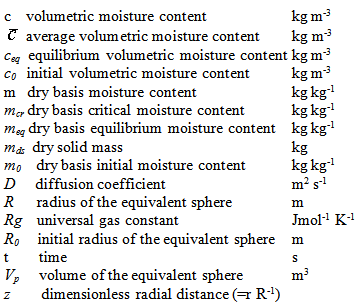References

  Demiray E., Tulek Y. Thin-layer drying of tomato slices in a convective hot air dryer. Heat and Mass Transfer, 48, 841-847. 2012.In article View Article  Hawlader M. N. A., Uddin M. S., Ho J. C., Teng A. B. W. Drying characteristics of tomatoes, Journal of Food Engineering, 14, 259-268. 1991.In article View Article  Giovanelli G., Zanoni B., Lavelli V., Nani R. Water Sorption, drying and antioxidant properties of dried tomato products, Journal of Food Engineering, 52, 135-141. 2002.In article View Article  Resende O., Correa P. C., Jarén C., Moure A. J. Bean moisture diffusivity and drying kinetics: a comparison of the liquid diffusion model when taking into account and neglecting grain shrinkage. Spanish Journal of Agricultural Research, 5 (1), 51-58. 2007.In article View Article  Crank J. The Mathematics of Diffusion, 2nd Edition, Clarendon Press, Oxford, 1975.In article  Crank J. Free and Moving Boundary Problems, Clarendon Press, Oxford, 1984.In article  Aguerre R. J., Tolaba M., Suarez C. Modeling volume changes in food drying and hydration, Latin American Applied Research, 38, 345-349. 2008.In article  Rivera B. D. Evaluación de la deshidratación de jitomate (Licopersicum Esculentum) con atemperado en lecho vibrofluidizado, considerando la retención de licopeno como parámetro de calidad, Master´s thesis. Escuela Nacional de Ciencias Biológicas, Instituto Politécnico Nacional, México, 2007.In article  Lewicki P. P., Le H. V., Pomaranska-Lazuka W. Effect of pretreatment on convective drying of tomatoes. Journal of Food Engineering, 54, 141-146. 2002.In article View Article  Kunii D., Levenspiel O. Fluidization Engineering, Second edition, Butterworth-Heinemann, USA, 1991.In article  Press W. H., Teukolsky S. A., Vetterling W. T., Flannery B. P. Numerical Recipes: The art of Scientific Computing, Third edition, Cambridge University Press, New York, USA, 2007.In article  Kingsly A. R. P., Singh R., Goyal R. K., Singh D. B. Thin-Layer Drying Behaviour of Organically Produced Tomato, Am. Journal of Food Technology, 2, 71-78. 2007.In article View Article  Liu X., Chen J., Liu M., Li Z., Tao Y., Zhu D. “The Effect of Biological Material’s Tissue Shrinking on Moisture Diffusivity during Hot-air-drying”. Proceedings of the World Automation Congress, Kobe, Japan, 2010, Pages 255-260.In article  Doymaz I. Air-drying characteristics of tomatoes, Journal of Food Engineering, 78, 1291-1297. 2007.In article View Article  Abano E., Ma. H, Wu. Q. Influence of Air Temperature on the Drying Kinetics and Quality of Tomato Slices, Journal of Food Processing and Technology, 2. 2011.In article  Chaijan, A. R., Parian A. J., Esna-Ashari M. Modeling of moisture diffusivity, activation energy and bspecific energy consumption of high moisture corn in a fixed and fluidized bed convective dryer. Spanish Journal of Agricultural Research, 9 (1), 28-40. 2011.In article View Article  Taiwo A., C., Sikiru A. R., Ojo A. Drying characteristics and sorption isotherm of tomato slices. Journal of Food Engineering, 73, 157-163. 2006.In article View Article  Zogzas N, P., Maroulis Z. D., Marinos-Koulis D. Moisture Diffusivity Data Compilation in Food Stuffs. Drying Technology, 14 (10), 2225-2253. 1996.In article View Article  Purkayastha M. D., Nath Amit, Deka B. Ch., Mahanta Ch. L. Thin layer drying of tomato slices. J. Food Sci. Technol., 50 (4), 642-653. 2013.In article View Article  PubMed